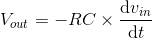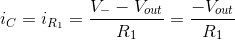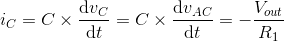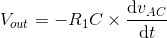# Differentiator Amplifier

## Working principle

The differentiator op-amp configuration produces an output voltage that is proportional to the rate of change of the input voltage by measuring the current through a capacitor:The right-hand side of the capacitor is held at 0 volts due to the virtual ground effect. Therefore, current through the capacitor is solely due to change in the input voltage. A steady input voltage won’t cause a current through C, but a changing input voltage will. The faster the voltage changes, the larger the magnitude of the output voltage.

### Formula derivation

Because of virtual ground and the op-amp’s infinite impedance, all current flowing through the capacitor also flows through R1:vc = vAC - icR2 ~ vAC (the very small resistance R2 is needed for convergence purposes)An application for this circuit could be monitoring the rate of change of temperature in an environment where too high or too low of a temperature rise is detrimental and would, thus, trigger an alarm or a notification using additional circuitry on the output.

### Experiment

• Set the AC voltage source’s amplitude and frequency to 0 and the DC voltage source’s value to 1 V. Observe the output after there is no change in the input voltage – after the capacitor C charges up, do you see what you expected? If the input voltage is constant, dv/dt is zero and the output voltage is zero.